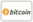Bookcover of Denormal Number
Booktitle:

# Denormal Number

## Computer science, Normal number (computing), Arithmetic underflow, Floating point, IEEE 754-2008

Miss Press (2011-05-19 )eligible for voucher
ISBN-13:

### 978-613-5-80471-3

ISBN-10:
6135804715
EAN:
9786135804713
Book language:
English
Blurb/Shorttext:
Please note that the content of this book primarily consists of articles available from Wikipedia or other free sources online. In computer science, denormal numbers or denormalized numbers fill the underflow gap around zero in floating point arithmetic: any non-zero number which is smaller than the smallest normal number is 'sub-normal'. For example, if the smallest positive 'normal' number is 1×β−n, then any smaller positive numbers that can be represented are denormal. The significand of an IEEE number is the part of a floating point number that represents the significant digits. For a positive normalised number it can be represented as m0.m1m2m3...mp-2mp-1. Notice that for a binary radix, the leading binary digit is one. In a denormal number, since the exponent is the smallest that it can be, zero is the lead significand digit in order to represent numbers closer to zero than the smallest normal number.
Publishing house:
Miss Press
Website:
http://www.alphascript-publishing.com
Edited by:
Niek Yoan
Number of pages:
60
Published on:
2011-05-19
Stock:
Available
Category:
Informatics, IT
Price:
29.00 €
Keywords:
Computer, Science, Number, Arithmetic, Floating### Categories